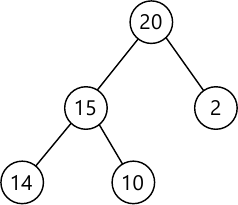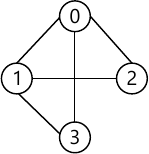MORE IN Data Structures and Applications
VTU Computer Science (Semester 3)
Data Structures and Applications
June 2013
Total marks: --
Total time: --
INSTRUCTIONS
(1) Assume appropriate data and state your reasons
(2) Marks are given to the right of every question
(3) Draw neat diagrams wherever necessary

1 (a) Define pointer. With example, explain pointer declaration, pointer initialization and use of the pointer in allocating a block of memory dynamically.
6 M
1 (b) Define recursion. Give two conditions to be followed for successive working of recursive program. Given recursive implementation of binary's search with property commnets.
6 M
1 (c) Define three asymptotic notations and give the asymptotic representation of function 3n+2 in all the three notation and prove the same from first principle method.
8 M

2 (a) What is a structure? Give three different ways of defining structure and declaring variables and method of accessing member of structure using a student structure with roll number, name and marks in 3 subjects as members of that structure as example.
6 M
2 (b) Give ADT sparse matrix and show with a suitable example sparse matrix representation storing as triples. Give simple transpose function to transpose sparse matrix and give its complexity.
8 M
2 (c) How would you represent two sparse polynomials using array of structure and also write a function to add that polynomial and store the result in the same array.
6 M

3 (a) Give ADT stack and with neacessary function explain implementing stacks to hold records with different types of field in stack.
6 M
3 (b) Give the disadvantage of ordinary queue and how it is solved in circular queue. Explain the same. Explain with suitable example how would you implement circular queue using dynamically allocated arrays.
8 M
3 (c) Convert the infix expression a/b-c+d*e-a-c into postfix expression. Write a function to evaluate that postfix expression and trace that for given data a=6, b=3, c=1, d=2, e=4.
6 M

4 (a) Give the mode structure to create a linked list of integers and write C functions to perform the following :-
i) Create a three-node list with data 10, 20 and 30
ii) Insert a node with data value 15 in between the nodes having data values 10 and 20
iii) Delete the node which is followed by a node whose data value is 20
iv) Display the resulting singly linked list.
8 M
4 (b) With node structure show how would you store the polynomials in linked lists? Write C function for adding two polynomials represented as circular lists.
6 M
4 (c) Write a notes on:
i) Linked representation of sparse matrix
6 M

5 (a) Define a binary tree and with example show array representation and linked presentation of binary tree.
6 M
5 (b) Write an expression tree for an expression A/B+C*D+E. Give the algorithm for inorder, postorder and preorder traversals and apply that traversal method to the expression tree and give the result of transversals.
8 M
5 (c) Define a Max Heap. Explain clearly inserting an element that has value 21 for the heap shown in Fig.Q5(c), given below and show the resulting heap.6 M

6 (a) Define a binary search tree and construct a binary search tree. With elements (22,28,20,25,22,15,18,10,14). Give recursive search algorithm to search an element in that tree.
6 M
6 (b) What is a winner tree? Explain with suitable example a winner tree for k=8
6 M
6 (c) Construct a binary tree having the following sequences.
i) Preorder sequence: ABCDEFGHI
ii) Inorder sequence: BCAEDGHFI
Show the steps if constructing binary tree in the above example.
3 M
6 (d) Give the adjauncy matrix and adjacinty lists representation for the graph shown in Fig.Q6(d).5 M

7 (a) Define the following:
i) Single ended priority queues
ii) Double ended priority queues
iii) Height-based leftist trees
iv) Weight-based leftist trees
v) A binomial tree
vi) Extended binary tree.
6 M
7 (b) With suitable example, explain leftist trees and give structures of nodes.
6 M
7 (c) What is Fibonacci heap? Give suitable example and give the steps for deletion of node and decrease key of specified node in F-heap.
8 M

8 (a) What is an AVL tree? Starting with an empty AVL tree perform the following sequence of insertions. MARCH, MAY, NOVEMBER, AUGUST, APRIL, JAUNARY , DECEMBER, JULY, FEBRUARY, DRAW the AVL tree following each insertion and state rotation type if any for any insert operation.
10 M
8 (b) Define RED-BLACK trees and give its additional properties starting with an empty red-block tree insert the following keys in the given order { 50,10,80,90,70,60,65,62}. Giving color changing and rotation instances.
10 M

More question papers from Data Structures and Applications